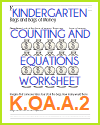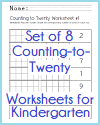﻿
Kindergarten: Number and Operations in Base Ten | Student HandoutsKindergarten Math: Number & Operations in Base Ten
Kindergarten > Kindergarten Mathematics > Kindergarten Number and Operations in Base Ten

CCSS.MATH.CONTENT.K.NBT.A.1 - Compose and decompose numbers from 11 to 19 into ten ones and some further ones, e.g., by using objects or drawings, and record each composition or decomposition by a drawing or equation (such as 18 = 10 + 8); understand that these numbers are composed of ten ones and one, two, three, four, five, six, seven, eight, or nine ones.Bags of Money Writing an Equation Worksheet Shade Numbers to Twenty; Set of 8 Worksheets